# How to Convert Date & Time Format to Date in Excel

Sometimes we want to convert date and time format to date only format in excel for example convert `01/29/2019 06:51:03` to `01/29/2019`, we can convert format by Formula or Format Settings. The two ways are easy to learn, so you can select one you like to convert date and time format.

## Convert Date & Time to Date by Formula

Prepare a table with date and time, the format is `MM/DD/YYYY HH/MM/SS`.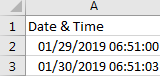If we want to convert them to date format, we can follow below steps:

Step 1: In B2, enter the formula =MONTH(A2) & “/” & DAY(A2) & “/” & YEAR(A2).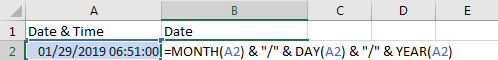Step 2: Click Enter to get the result. Verify that format is changed to date properly, time part is cleared.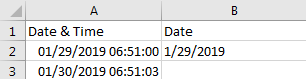Step 3: Drag the Fill Handle down to fill B3. Verify that B3 is changed to date format properly.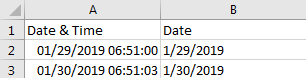## Convert Date & Time to Date by Format Settings

Step 1: On A2, right click to load menu, select Format Cells.Step 2: In Format Cells window, under Number tab, in Category list, select Date; then in Type list select one format you want to show the date. Then the date format is displayed in Sample field.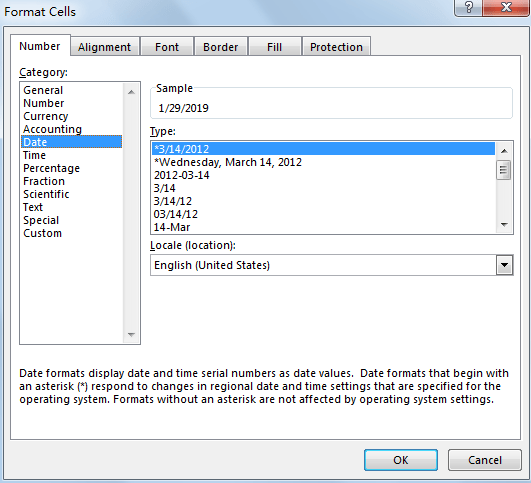Step 3: Click OK. Verify that previous date and time is changed to date format.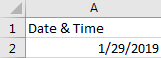### Related Functions

• Excel YEAR function
The Excel YEAR function returns a four-digit year from a given date value, the year is returned as an integer ranging from 1900 to 9999. The syntax of the YEAR function is as below:=YEAR (serial_number)…
• Excel MONTH function
The Excel MONTH function returns the month of a date represented by a serial number. And the month is an integer number from 1 to 12. The syntax of the MONTH function is as below:=MONTH (serial_number)…
• Excel DAY function
The Excel DAY function returns a day of a date (from 1 to 31).The DAY function is a build-in function in Microsoft Excel and it is categorized as a DATE and TIME Function.The syntax of the DAY function is as below:= DAY (date_value)…

Related Posts

Calculating Average Of The Numbers

Are you weary of investing a lot of time and effort in manually calculating the average of the numbers by including or excluding 0 and calculating the average of the top 3 scores? Then congratulations because you have just landed ...

Add Months To Date In Excel

It is important to adjust time periods when performing financial modeling. In Excel, you can use the MONTH function to add a specific number of months to a date. The MONTH function takes one argument: the number of months you ...

Add Days to Date in MS Excel

If you are an vavid MS Excel user, then you might have come across situations where you need to add the same or different days into the particular date, and if you have done this task manually, then let me ...

Filter Data By Date Field

The use of Microsoft Excel's Format Cells and Auto Filter option is a great way to filter data by year or Month. This article will discuss how to filter by date field using Filter function and Format cells in Microsoft ...

How to Count Dates of Given Year in Excel

This post will guide you how to count Dates of a certain year in the range of dates using a formula in Excel 2013/2016 or Excel office 365. How do I count dates by a given year in Excel. And ...

How to Sum Values Based on Month and Year in Excel

We often do some summary or statistic at the end of one month or one year. In these summary tables, there are at least two columns, one column records the date, and the other column records the sales or product ...

How to Extract Year from Date & Time Format in Excel

Sometimes we want to get Year information from date and time format to show the Year only in excel. For example convert 01/29/2019 06:51:03 to 2019, we can get Year information by Formula or Format Settings. The two ways are ...

How to Calculate Remaining Days in a Month or Year in Excel

This post will guide you how to calculate remaining days in a given month or year in Excel. How do I calculate the number of days left in a month or year using a formula in Excel 2013/2016. Calculate Remaining ...

How to Split Date into Day, Month and Year in Excel

This post will guide you how to split date into separate day, month and year in excel. How do I quickly split date as Day, Month and Year using Formulas or Text to Columns feature in Excel. Split Date into ...

How to sort Dates by Month and Day Only in Excel

This post will guide you how to sort dates or birthdays by month or day only in Excel. How do I sort dates by Year or Month or Day only with a formula in Excel 2013/2016. How to use the ...

Sidebar